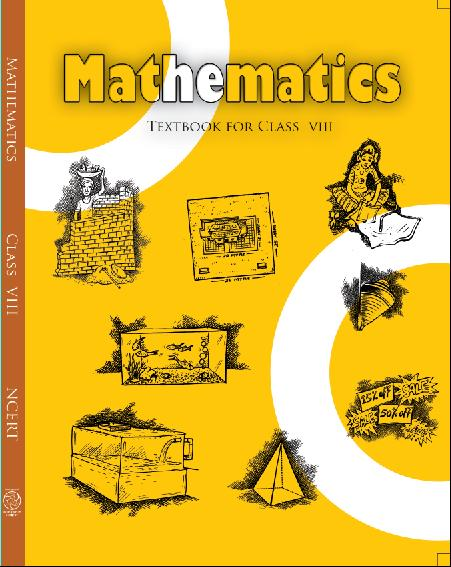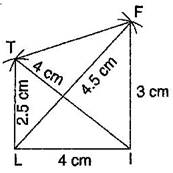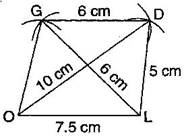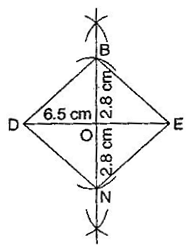1. /
2. CBSE
3. /
4. Class 08
5. /
6. Mathematics
7. /
8. NCERT Solutions for Class...

# NCERT Solutions for Class 8 Maths Exercise 4.2### myCBSEguide App

Download the app to get CBSE Sample Papers 2023-24, NCERT Solutions (Revised), Most Important Questions, Previous Year Question Bank, Mock Tests, and Detailed Notes.

NCERT solutions for Class 8 Maths Practical Geometry## NCERT Solutions for Class 8 Maths Practical Geometry

###### 1. Construct the following quadrilaterals:

LI = 4 cm, IF = 3 cm, TL = 2.5 cm, LF = 4.5 cm, IT = 4 cm

OL = 7.5 cm, GL = 6 cm, GD = 6 cm, LD = 5 cm, OD = 10 cm

(iii) Rhombus BEND

BN = 5.6 cm, DE = 6.5 cm

Ans.

NCERT Solutions for Class 8 Maths Exercise 4.2

###### (i)Given:  LI = 4 cm, IF = 3 cm, TL = 2.5 cm, LF = 4.5 cm, IT = 4 cm

Steps of construction:(a) Draw a line segment LI = 4 cm.

(b) Taking radius 4.5 cm, draw an arc taking L as centre.

(c) Draw an arc of 3 cm taking I as centre which intersects the first arc at F.

(d) Join FI and FL.

(e) Draw another arc of radius 2.5 cm taking L as centre and 4 cm taking I as centre which intersect at T.

(f) Join TF, Tl and TI.

It is the required quadrilateral LIFT.

NCERT Solutions for Class 8 Maths Exercise 4.2

###### (ii)Given:  OL = 7.5 cm, GL = 6 cm, GD = 6 cm, LD = 5 cm, OD = 10 cm

Steps of construction:(a) Draw a line segment OL = 7.5 cm

(b) Draw an arc of radius 5 cm taking L as centre and another arc of radius 10 cm taking O as centre which intersect the first arc point at D.

(c) Join LD and OD.

(d) Draw an arc of radius 6 cm from D and draw another arc of radius 6 cm taking L as centre, which intersects at G.

(e) Join GD and GO.

It is the required quadrilateral GOLD.

NCERT Solutions for Class 8 Maths Exercise 4.2

###### (iii)Given:  BN = 5.6 cm, DE = 6.5 cm

To construct: A rhombus BEND.

Steps of construction:(a) Draw DE = 6.5 cm.

(b) Draw perpendicular bisector of line segment DE.

(c) Draw two arcs of radius 2.8 cm from intersection point O, which intersects the line KN at B and N.

(d) Join BE, BD as well as ND and NE.

It is the required rhombus BEND.

## NCERT Solutions for Class 8 Maths Exercise 4.2

NCERT Solutions Class 8 Mathematics PDF (Download) Free from myCBSEguide app and myCBSEguide website. Ncert solution class 8 Mathematics includes text book solutions from Class 8 Maths Book . NCERT Solutions for CBSE Class 8 Maths have total 16 chapters. 8 Maths NCERT Solutions in PDF for free Download on our website. Ncert class 8 solutions PDF and Maths ncert class 8 PDF solutions with latest modifications and as per the latest CBSE syllabus are only available in myCBSEguide.

## CBSE app for Class 8

To download NCERT Solutions for class 8 Social Science, Computer Science, Home Science,Hindi ,English, Maths Science do check myCBSEguide app or website. myCBSEguide provides sample papers with solution, test papers for chapter-wise practice, NCERT solutions, NCERT Exemplar solutions, quick revision notes for ready reference, CBSE guess papers and CBSE important question papers. Sample Paper all are made available through the best app for CBSE students and myCBSEguide website.Test Generator

Create question paper PDF and online tests with your own name & logo in minutes.myCBSEguide

Question Bank, Mock Tests, Exam Papers, NCERT Solutions, Sample Papers, Notes

### 8 thoughts on “NCERT Solutions for Class 8 Maths Exercise 4.2”

1. Nice it is easy to study than fair note it is very useful for any doubt. Very thanks .

Tomorrow maths exam …..

It will be very useful

2. its verygood, usely our future

3. Super

4. Nice Web site l got all answer
Thanks for making a wonderful Web site ??
This is best website ????

5. Wowww

6. Wow

7. Nice

8. Thank you for your help ????